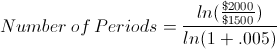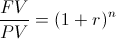# Solve for Number of Periods - PV & FVThe formula for solving for the number of periods shown at the top of this page is used to calculate the length of time required for a single cash flow(present value) to reach a certain amount(future value) based on the time value of money. In other words, this formula is used to calculate the length of time a present value would need to reach the future value, given a certain interest rate.

The formula for solving for number of periods may also be referred to as solving for n, solving for term, or solving for time. Solving for n originates from the present value and future value formulas in which the variable n denotes the number of periods.

It is important to keep in mind that the number of periods and periodic rate should match one another. For example, if the rate is compounded monthly, then the monthly rate would be used and the number of periods would reflect the number of months.

## Example of Solve for Number of Periods Formula (PV & FV)

An example of solving for the number of periods formula would be an individual who would like to determine how long it would take for his \$1500 balance in his account to reach \$2000 in an account that pays 6% interest, compounded monthly. Of course, for this example it is assumed that there will be no deposits nor withdrawals within this timeframe.

As previously stated in the prior section, the number of periods and the periodic rate should match one another. The 6% annual interest rate is compounded monthly, so .005(equal to .5%) would be used for r as this is the monthly rate.

For this example, the equation to solve for the number of periods would bewhich would result in 57.68 months. Of course in real situations the fraction of a month may not be exact due to when the account is credited, there may be charges to the account that must be accounted for, and so on.

This can be checked by putting these variables into the present value formula and confirming that in fact there will be a \$2000 balance after 57.68 months based on a monthly rate of .5%.

## How is the Formula for Number of Periods Derived?

The formula to calculate the number of periods based on present value and future value can be found by first looking at the future value formula ofThe first step is to divide both sides by PV which would show asFrom here we will use logarithms and take the ln of both sides which would showFrom here, both sides of this formula can be divided by ln(1+r) which will equal the formula shown at the top of this page.

New to Finance?